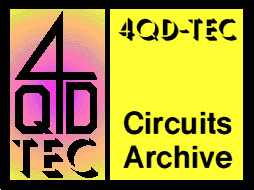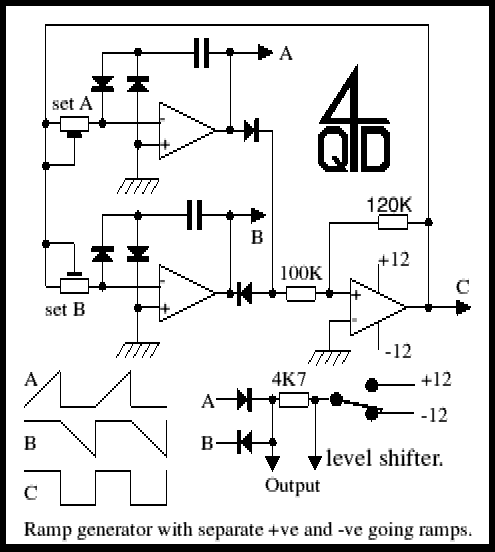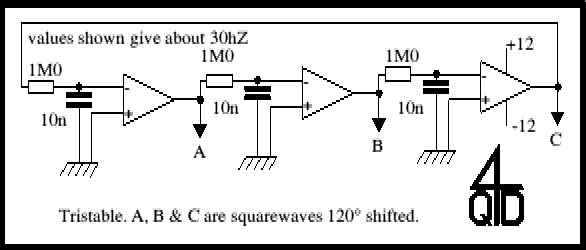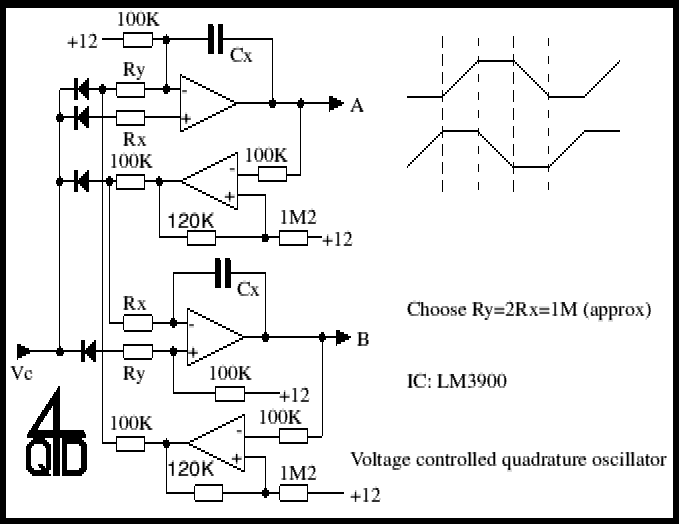# 4QD-TEC: Electronics Circuits Reference Archive Waveform generator circuits

There are many different types of waveform generator circuits for many different uses. Here are some which I've collected. I leave it up to you to find uses for them!

### Dual Ramp GeneratorThe first circuit is a dual ramp generator where the positive and negative ramps are generated separately. This circuit was used as a ramp generator for a transistor curve tracer: the positive going ramp was used for testing NPN transistors and the negative ramp for testing PNP transistors. The two ramp outputs are at A and B. The A output sits at -12v whilst the B output is ramping and then it ramps positive while the B output sits at +12v. The two waveforms are shown below the circuit and ramp lengths are determined by the integrator (-ve feedback) capacitors and the 'set' presets. For transistor testing this is nice since each ramp is 50% of the duty cycle so the transistor gets a chance to rest between ramps. If the output is selected with respect to +ve or negative (which requires an isolated supply) the polarity can be switched as shown in the bottom right of the diagram.

### Three phase oscillatorThe second circuit is a 3 phase squarewave oscillator, or tristable. Its operation should be reasonably obvious and it is like a simple bistable oscillator with 3 stages.

### Quadrature (sine/cosine) voltage controlled oscillatorThis is a voltage controlled oscillator with a difference: it gives a quadrature output which is shaped as shown in the diagram, with an output which approximates to a sine/cosine waveform. If you have difficulty understanding it this is because it does not use standard op-amps but an LM3900 which is a Norton op-amp - it works on differential currents, not on differential voltages. The LM3900 is a very versatile op-amp which has some very special advantages over standard op-amps but is not nearly as commonly used: I suspect that few students are taught about it so few consider using it.